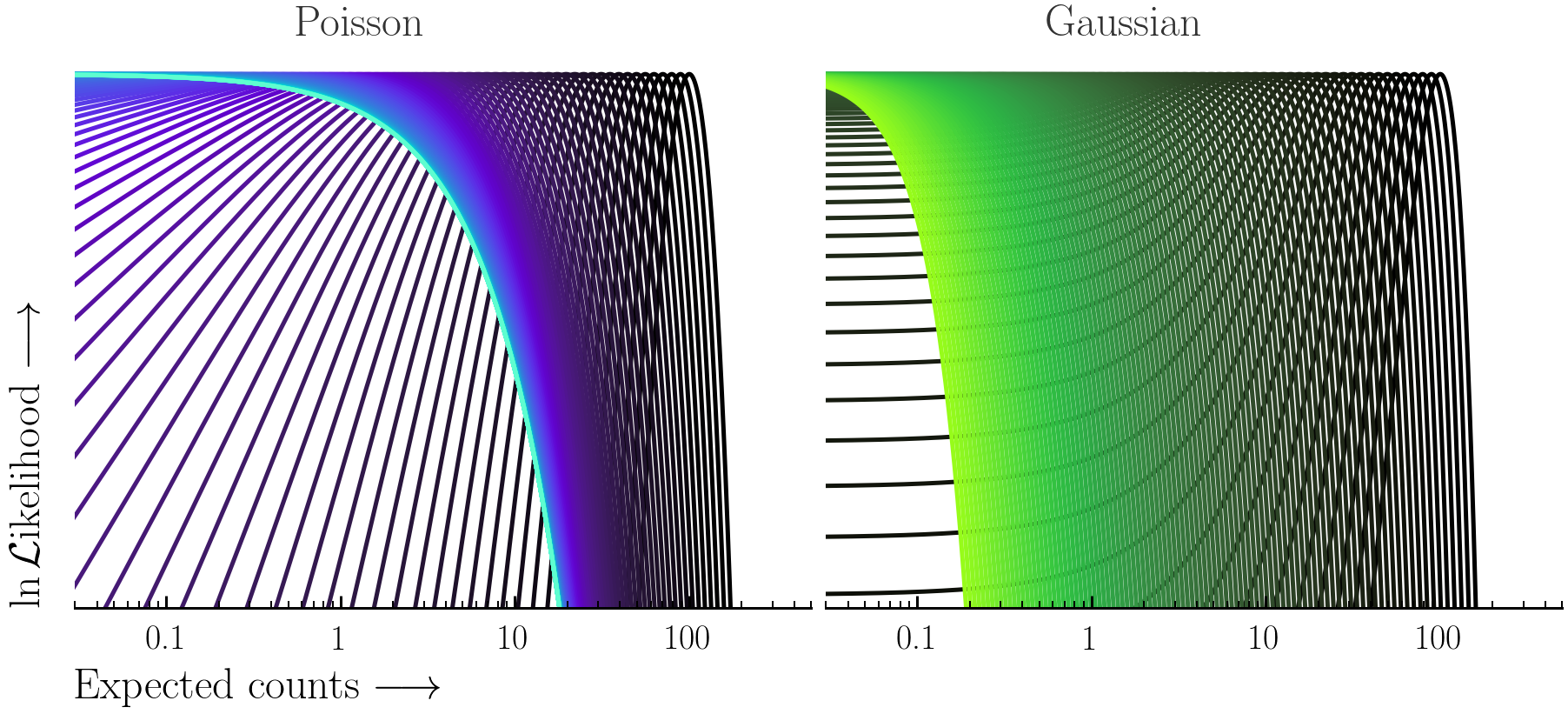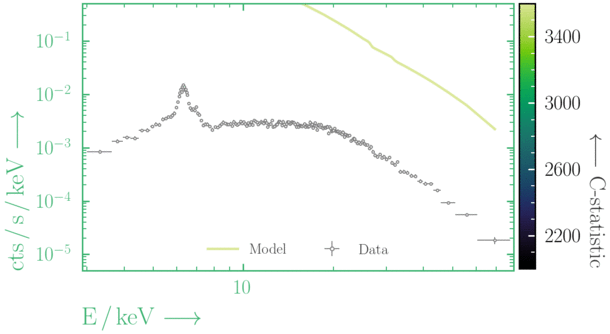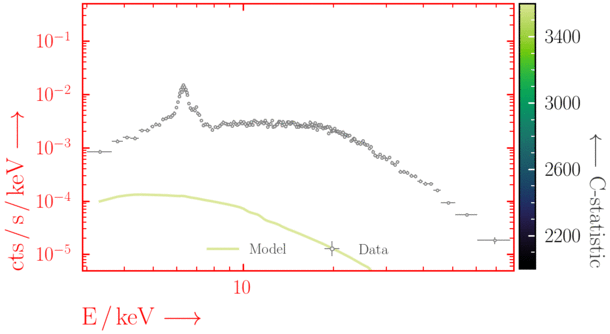## Session 0 - X-ray spectral fitting

This session aims to provide a sufficient background with Xspec/PyXspec and/or Sherpa to complete the remaining BXA tutorial exercises. For a complete and in-depth understanding of the features available in these software packages, see the useful links for the excellent online material provided by each team.

Key objectives:
• Load a spectrum and fit a model to it in Xspec, PyXspec and/or Sherpa
• Manually explore a parameter space and avoid local minima
• Get to grips with plotting in Xspec, PyXspec and Sherpa

### Getting started

If you don't have your own, download the following NuSTAR spectral files from here. The zip file contains:
• Source spectrum (binned with `ftgrouppha grouptype=bmin groupscale=5`): `nustar_src.pha`
• Response: `nustar.rmf`
• Effective area: `nustar.arf`
• Background spectrum: `nustar_bkg.pha`
Here are some initial steps to set up a fit for BXA in PyXspec or Sherpa:
• For interactive mode:
• Xspec:
`\$ xspec`
• PyXspec:
```\$ ipython from xspec import * ```
• Sherpa:
`\$ sherpa`

• For scripting:
• Xspec:
`\$ xspec - xspec_script.tcl`
Remember to include `exit` or `quit` at the end of the script!
• PyXspec:
`\$ python3 pyxspec_script.py`
• Sherpa:
`\$ sherpa sherpa_script.py`

### Exercise 0.1 - binning data

• The majority of the exercises in this tutorial use the Wstat fit statistic during fitting, which adds a background contribution to the source model in each channel. If an insufficient fraction of background bins contain an insufficient number of counts, using Wstat can lead to biased results. In such scenarios, the background is under-estimated, leading to the source flux being over-estimated. For more details on this bias, see the blog post by Giacomo Vianello here

• To avoid this issue, it is beneficial to bin the source + background spectrum enough so that the background (which is automatically binned to match the source + background spectrum in e.g., Xspec) spectral bins contain enough counts. Some popular options for binning using the ftool `ftgrouppha` include:
• `grouptype=opt`: uses the optimal binning scheme of Kaastra & Bleeker (2016; A&A 587, A151).
• `grouptype=bmin`: binning to have a minimum number of counts in each background bin.
• `grouptype=min`: binning to have a minimum number of counts in each source + background spectrum bin.

• The solutions for these exercises were produced using `bmin=5` with the following `ftgrouppha` script (make sure to be inside the directory containing the spectral files):
```ftgrouppha grouptype=bmin groupscale=5 clobber=yes \ infile=nustar_src.pha \ backfile=nustar_bkg.pha \ outfile=nustar_src_bmin5.pha ```

• If using a binned spectrum in a fit (i.e. there is no background model), there is often no single option that is ideal for all scenarios. It is recommended to try a variety of different methods to ensure biases are minimal in a fit.

Before starting, the following commands are required:
• Set the x-axis units (e.g., instrument channels or energy):
• Xspec:
`setplot energy`
• PyXspec:
`Plot.xAxis = "keV"# or "channel"`
• Sherpa:
`set_analysis("ener")# or "chan"`

• Plot device (Xspec & PyXspec only):
• Xspec:
• Set the plot device: `cpd /xw`
• PyXspec:
• No plot device (useful to avoid plot window pop-ups): `Plot.device = "/null"`
• Set the plot device: `Plot.device = "/xw"`
(other plot devices are available, see here)

Next, we load in the data and ignore ineffective energies:
• Xspec:
`data 1:1 nustar_src_bmin5.pha`
• PyXspec:
`s = Spectrum("nustar_src_bmin5.pha")`
Or:
`AllData("1:1 nustar_src_bmin5.pha")`
• Sherpa:
`load_pha(1, "nustar_src_bmin5.pha")`

• Ignore ineffective energies:
• Xspec:
`ignore 1:0.-3. 79.-**`
• PyXspec:
`s.ignore("0.-3. 79.-**")`
Or:
`AllData.ignore("1:0.-3. 79.-**")`
• Sherpa:
`ignore_id(1, "0.:3.,79.:")`

### Exercise 0.3 - plotting

There are a number of useful settings for plotting the data in Xspec & PyXspec. For a full list, see here. Some useful examples for these exercises include:
• Xspec:
• `setplot area`/`noarea`:
For plots, divide the observed data by the response effective area
• `setplot add`/`noadd`:
Show all additive model components in plots
• `setplot background`/`nobackground`:
Automatically plot the background in the same plot as the source
• `setplot rebin X Y N`:
Adjacent bins are combined in dataset `N` until either: (1) the significance of the combined bins is ≥ X σ or (2) `Y` bins have been combined. To undo on all datasets, use `setplot rebin 0 1 -1`.
• `setplot command range x xmin xmax`
To append any IPLOT commands to a list that is executed when the user types `plot ... `.
• `setplot delete all`
To delete all IPLOT commands from the current list.
• PyXspec (for command descriptions see above, for command documentation see here):
• `Plot.area=True`/`False`
• `Plot.add=True`/`False`
• `Plot.background=True`/`False`
• `Plot.setRebin(X, Y, N)`
• `Plot.addCommand("range x xmin xmax")`
• `Plot.commands = ()`
Plot the observed data inside Xspec, PyXspec and Sherpa:
• Xspec (note you can try `counts`/`data`/`lcounts` in place of `ldata`):
```setplot rebin 2 1000 -1 plot ldata ```
• PyXspec (note you can try `"counts"`/`"data"`/`"lcounts"` in place of `"ldata"`):
```Plot.setRebin(2, 1000) Plot("ldata") ```
• Sherpa (note you can try `"counts"` in place of `"rate"`):
```group_snr(1, 2.) subtract(1) set_analysis(1, "ener", "rate") plot_data(xlog=True, ylog=True) ```

#### Interactive `PLT` (Xspec only)

In Xspec, you can either use `plot` or `iplot` to produce plots. `plot` will make the plot and return the user to the Xspec prompt, whereas `iplot` starts the interactive plotting interface which can be used to edit a plot.
You can always view the full list of PLT commands being run by Xspec if you increase the verbosity to `chatter 25` before typing `iplot`. This is useful for determining the plotting element numbers for different colours, etc. Here are some basic commands for using the interactive PLT interface:
• `LAB title text`: Change the title of the plot to "text"
• `time off`: Remove the time label in the bottom right of the plot
• `CS N`: Change the character size for text on the plot (values of 0-5 are allowed, 1 is default)
• `viewport xlo ylo xhi yhi`: Change the location of the plot in the plotting window
• `LAB y ylabel`: Change the y axis label
• `LAB x xlabel`: Change the x axis label
• `COL 0 on 1..2`: Change the colour of elements 1..2 to 0 (i.e. black - for all available colours, see Fig. 5.1 here)
• `LW 5 on 1..2`: Change the line width of elements 1-2 to 5 (values can be ≥ 1)
• `LAB 5 POS x y "This text is... \fiITALIC\fn NORMAL\uSUPERSCRIPT\d\dSUBSCRIPT"`: create a text label.
• Greek alphabet in order (use `\G` for capitals):
`\ga \gb \gg \gd \ge \gz \gy \gh \gi \gk \gl \gm \gn \gc, \go, \gp, \gr \gs \gt \gu \gf \gx \gq \gw`
• `LAB 5 COL 4 CS 1.4`: Change the colour of label 5 to 4 (i.e. blue) and change the character size to 1.4
IPLOT commands can be used from the Xspec prompt by appending all the commands to a list. Xspec then runs these commands only when you type `plot`. To interact with this list of commands:
• `setplot command ...`: Type this followed by any interactive `PLT` command to append the command to the list
• `setplot list`: Show the currently appended plot commands
• `setplot delete all`: Remove all plot commands from the list
A useful tip is to use the command `wdata filename.dat` inside interactive `PLT` (or use `setplot command wdata filename.dat` with Xspec `plot`) to save everything being plotted by Xspec to `filename.dat` (make sure to keep track of the order of the columns!). This file can then be read in with e.g., `pandas` to plot in Python.

Saving the data to be plotted by yourself can be done with the following:
• Xspec:
```setplot rebin 2 1000 -1 setplot command wdata ldata_plot.dat plot ldata exit ```
Then the data can be read in to e.g., `pandas` with: ```import pandas as pd df = pd.read_csv("ldata_plot.dat", skiprows=3, delim_whitespace=True, names=["EkeV", "EkeV_err", "data", "data_err"]) ```
• PyXspec:
```import pandas as pd Plot.setRebin(2, 1000) EkeV = Plot.x() EkeV_err = Plot.xErr() data = Plot.y() data_err = Plot.yErr() df = pd.DataFrame(data={"EkeV": EkeV, "EkeV_err": EkeV_err, "data": data, "data_err": data_err}) df.to_csv("csv_filename.csv", index=False) ```
• Sherpa:
```import pandas as pd group_snr(1, 2.) subtract(1) set_analysis(0, "ener", "rate") srcdata = get_data_plot(1) EkeV = srcdata.x EkeV_err = srcdata.xerr data = srcdata.y data_err = srcdata.yerr df = pd.DataFrame(data={"EkeV": EkeV, "EkeV_err": EkeV_err, "data": data, "data_err": data_err}) df.to_csv("csv_filename.csv", index=False) ```
Save the data being plotted and produce your own spectral plots in the plotting package of your choice. If using Python, here is an example to get started:
```import matplotlib.pyplot as plt fig, ax = plt.subplots() err_kwargs = {} err_kwargs["fmt"] = "o" err_kwargs["label"] = "Folded data" err_kwargs["mec"] = "royalblue" err_kwargs["ecolor"] = "royalblue" err_kwargs["mfc"] = "white" err_kwargs["markersize"] = 6. err_kwargs["markeredgewidth"] = 1. err_kwargs["linewidth"] = 1. err_kwargs["capthick"] = 0. err_kwargs["capsize"] = 0. err_kwargs["zorder"] = -1. ax.errorbar(df["EkeV"], df["data"], xerr=df["EkeV_err"], yerr=df["data_err"], **err_kwargs) ax.set_xscale("log") ax.set_yscale("log") plt.show() fig.savefig("folded_data.png") ```

### Exercise 0.4 - fit a model to data

The count rate that we detect and plot as a spectrum with e.g., `plot ldata` in Xspec is not the true spectrum, but a form of the true data that has been folded with all the effects arising from detecting the source. This is why fitting is done with forward-folding.
Forward-folding is the process creating a model from some parameters, and then convolving it with the detector response and effective area. The convolved version of the model is then compared with the convolved data by some statistical measure, and is repeated until the parameters are optimised according to the statistical measure.• Set the fit statistic (here we use modified Poisson statistics, which are appropriate in relatively low counts - see figure below):
• Xspec:
`set stat cstat`
• PyXspec:
`Fit.statMethod = "cstat"`
• Sherpa:
`set_stat("wstat")`

•Here the Poisson and Gaussian statistics only become comparable in the high-count regime.

• (Optional) turn off the interruption whilst fitting (Xspec & PyXspec only):
• Xspec:
`query yes`
• PyXspec:
`Fit.query = "yes"`

Then to start with, create a simple `powerlaw` model and set the model parameter values:
• Create the model:
• Xspec:
`model powerlaw`
• PyXspec:
`mymod = Model("powerlaw")`
• Sherpa:
```mymod = xspowerlaw.mypow set_source(1, mymod) ```
Note `xs` is used to indicate Xspec models, and get_default_id() can be used to show the id that is used if an id is not specified

• Set the parameter values:
Note the `min`, `max` parameter bounds are used in the BXA priors and should be set to reasonable values.
• Xspec:
```newpar 1 1.9, 0.1, -1., -1., 4., 4. newpar 2 1.e-3, 0.1, 1.e-8, 1.e-8, 1., 1. ```
• PyXspec:
```mymod.powerlaw.PhoIndex.values = (1.9, 0.1, -1., -1., 4., 4.) mymod.powerlaw.norm.values = (1.e-3, 0.1, 1.e-8, 1.e-8, 1., 1.) ```
• Or:
```AllModels(1)(1).values = 1.9, 0.1, -1., -1., 4., 4. AllModels(1)(2).values = 1.e-3, 0.1, 1.e-8, 1.e-8, 1., 1. ```
• Sherpa:
```set_par(mypow.phoindex, val=1.9, min=-1., max=4.) set_par(mypow.norm, val=1.e-3, min=1.e-8, max=1.) ```
Then we plot the data + model and fit (try to always plot before you fit! - this helps to see if everything is in order):
• Xspec:
```plot ldata del fit```
• PyXspec:
```Plot("ldata del") Fit.perform()```
• Sherpa (note if you subtracted the data previously with `subtract`, you need to run `unsubtract` if fitting with `wstat` or `cstat`):
```plot_fit(xlog=True, ylog=True) fit()```

#### Beware of local minima!

Depending on where you start your fit, the Levenberg-Marquadt algorithm may struggle to find the global minimum (i.e. the true best-fit).

In this example, both plots show an example progression for a fit with the Levenberg-Marquadt algorithm in Xspec. The final fit in the left panel is the global minimum, whereas the right hand plot is a local minimum. Local minima can be quite hard to identify visually and even masquerade as a very good fit. A quick way to initially check is to look for physically-unrealistic parameter values from a fit. The more thorough way to combat this issue whilst using Levenberg-Marquadt is to try to explore the parameter space more fully - e.g., with `steppar` in Xspec/PyXspec or by manually starting the fit from different initial parameter values.Have you found the best-fit parameter values for your `powerlaw` fit? Try starting the parameters at different initial values before fitting to check.

#### Plotting a model with data

When plotting a model with data, there are a few options inside Xspec, PyXspec and Sherpa:
• Folded units:
See the commands from Exercise 0.1
• Unfolded units:
Note that plotting in unfolded units is useful to see the possible unconvolved spectrum, but this is model dependent. Changing the model will change the plot.
• Xspec:
• `plot ufspec`
• `plot eufspec`
• `plot eeufspec`
• PyXspec:
• `Plot("ufspec")`
• `Plot("eufspec")`
• `Plot("eeufspec")`
• Note the model y-axis values can be accessed after running the `Plot(...)` command with e.g., `mod_data = Plot.model()`.
• Sherpa:
• Residuals:
• Xspec:
• `plot residuals`
Plot the data minus the folded model.
• `plot ratio`
Plot the data divided by the folded model.
• `plot delchi`
For `cstat`, this plots `(data-model)/error` where `error` is the square root of the model-predicted number of counts.
• PyXspec (see equivalent descriptions above):
• `Plot("residuals")`
• `Plot("ratio")`
• `Plot("delchi")`
• Sherpa:
• `plot_fit_resid(1)`
Plot the data and folded model together with the residuals.
• `plot_fit_ratio(1)`
Plot the data and folded model together with the ratio of model to data.
• `plot_fit_delchi(1)`
Plot the data and folded model together with the residuals.
• Plot your model fit and data with a residual method of your choice. What can you conclude?
Lastly, we grid over the parameter space to check for local minima:
• Xspec:
```steppar log/nolog parno1 lowerbound1 upperbound1 nsteps log/nolog parno2 lowerbound2 upperbound2 nsteps plot cont```
• PyXspec:
```Fit.steppar("log/nolog parno1 lowerbound1 upperbound1 nsteps log/nolog parno2 lowerbound2 upperbound2 nsteps") Plot("cont")```
• Note: you will have to choose the `lowerbound` and `upperbound` in `steppar` to encompass the parameter values.
• Sherpa:
See Region Projection in this Sherpa guide.

### Exercise 0.5 - simulating data

Some of the exercises in this tutorial involve simulating spectra. To do this you will need a model, response file (rmf), effective area file (arf) and (optionally) a background spectrum file:
• PyXspec (using `fakeit`):
```mymod = Model("powerlaw") mymod.powerlaw.PhoIndex.values = (1.9, 0.01, -3., -3., 3., 3.) mymod.powerlaw.norm.values = (1.e-3, 0.01, 1.e-8, 1.e-8, 1., 1.) fakeit_kwargs = {} fakeit_kwargs["response"] = "rmf_file_name" fakeit_kwargs["arf"] = "arf_file_name" fakeit_kwargs["background"] = "bkg_file_name" fakeit_kwargs["exposure"] = exposure fakeit_kwargs["correction"] = "1." fakeit_kwargs["backExposure"] = exposure fakeit_kwargs["fileName"] = "output_filename.pha" AllData.fakeit(1, FakeitSettings(**fakeit_kwargs)) ```
• Sherpa (using `fake_pha`):
```mymod = xspowerlaw.mypow set_par(mymod.phoindex, val = 1.9, min = -3., max = 3.) set_par(mymod.norm, val = 1.e-3, min = 1.e-8, max = 1.) load_pha(1, "nustar_src_bmin5.pha") #loading pre-exising source spectrum with rmf, arf & bkg present set_source(1, mymod) fakepha_kwargs = {} fakepha_kwargs["rmf"] = get_rmf() fakepha_kwargs["arf"] = get_arf() fakepha_kwargs["bkg"] = get_bkg() fakepha_kwargs["exposure"] = 30.e3 fake_pha(1, **fakepha_kwargs) save_pha(1, "output_filename.pha", clobber = True) ```